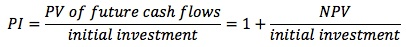#analyst-notes #cfa-level-1 #corporate-finance #has-images #reading-35-capital-budgeting #study-session-10

Profitability Index (PI)

This is an index used to evaluate proposals for which net present values have been determined. The profitability index is determined by dividing the present value of each proposal by its initial investment.The PI indicates the value you are receiving in exchange for one unit of currency invested.

• An index value greater than 1.0 is acceptable and the higher the number, the more financially attractive the proposal.
• A ratio of 1.0 is logically the lowest acceptable measure on the index. Any value lower than 1.0 would indicate that the project's PV is less than the initial investment.
If you want to change selection, open document below and click on "Move attachment"

Subject 3. Investment Decision Criteria
#13; Drawbacks: It does not take into account the time value of money; the value of cash flows does not diminish with time, as is the case with NPV and IRR. ARR is based on numbers that include non-cash items. <span>Profitability Index (PI) This is an index used to evaluate proposals for which net present values have been determined. The profitability index is determined by dividing the present value of each proposal by its initial investment. The PI indicates the value you are receiving in exchange for one unit of currency invested. An index value greater than 1.0 is acceptable and the higher the number, the more financially attractive the proposal. A ratio of 1.0 is logically the lowest acceptable measure on the index. Any value lower than 1.0 would indicate that the project's PV is less than the initial investment. Learning Outcome Statements d. calculate and interpret net present value (NPV), internal rate of return (IRR), payback period, discounted payback period, and profita